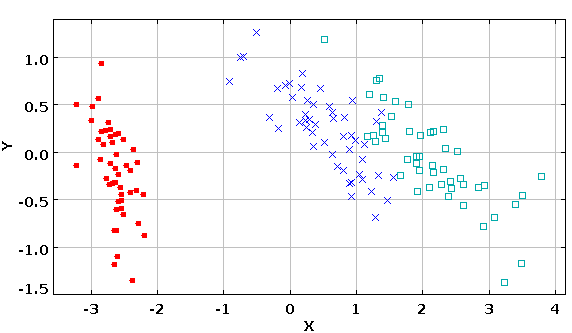# DMelt:Numeric/7 PCA Analysis

Limitted access. First login to DataMelt if you are a full DataMelt member. Then login to HandWiki as a user.

# PCA analysis

Principal Component Analysis (PCA) is an important for many applications. Read Principal_component_analysis.

Below we will show examples of Principal Component Analysis (PCA) data transformation using matrices as input. We will consider a situation when some of the columns in the data matrix are linearly dependent or when there are more columns than rows in the data matrix i.e. there are more dimensions than samples in the data set. In the above example we train the data and then apply to a test data.

The output of this code is shown below:

-0.9999999999999998, -0.5773502691896268
-0.08571428571428596, 1.732050807568878

Principal_component_analysis is used for Dimensionality_reduction, a method of transforming complex data in large dimensions into data with lesser dimensions ensuring that it conveys similar information.

It was described in [DMelt:Statistics/6_Dimensionality_reduction]. Let us consider IRIS dataset . The IRIS data set has 4 numerical attributes. Therefore, it is difficult for humans to visualize such data. Therefore, one can reduce the dimensionality of this dataset down to two. We will use Principal component analysis (PCA) which convert a set of observations of possibly correlated variables into a set of values of linearly uncorrelated variables called principal components. PCA needs the data samples to have a mean of ZERO, so we need a transform to ensue this property as well.

Here is the code that uses the Java packagejsat.datatransform.PCA to perform this transformation:

The output image is shown here:1. Fisher,R.A. "The use of multiple measurements in taxonomic problems", Annual Eugenics, 7, Part II, 179-188 (1936); also in "Contributions to Mathematical Statistics" (John Wiley, NY, 1950).